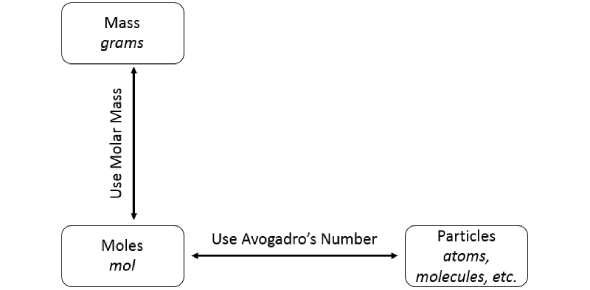# Mole Concept Trivia: Chemistry Quiz!

11 Questions | Total Attempts: 558Settings.

• 1.
What is Avogadro's number in scientific notation using the "e" with 3 sig figs?
• 2.
How many molecules are in 1 mole of water?
• 3.
How do chemists use the mole?
• A.

It is a way for scientist to measure things that are so small we can't see them

• B.

It is a way to make chemistry students hate chemistry

• C.

It is a way for scientist to measure very large molecules

• D.

It is a way for chemists to stay busy and get paid lots of money

• 4.
What is Avogadro's Number in regular notation with 3 sig figs? (no commas)
• 5.
Click all the possible units for molar mass.
• A.

Grams/mole

• B.

Mole/gram

• C.

Mol/g

• D.

G/mol

• E.

Grams

• F.

G

• G.

Grams per mole

• 6.
Define molar mass.
• A.

The mass, in grams, of 1 mole of a substance

• B.

The mass, in grams, of 1 mole of moles

• C.

Atomic mass

• D.

The mass of 1 atom

• 7.
What is the abbreviated/shortened way to write mole?
• 8.
The molar mass of hydrogen is 1.00794 grams.   How many hydrogen atoms are in 1.00794 grams of hydrogen?
• 9.
What scientist gets credit for coming up with the number of particles in 1 mole which is  6.0221415e23?  (from the LESSON -  Mole Concept)
• A.

• B.

Albert Einstein

• C.

Alexander Bell

• D.

Bill Nye the Science Guy

• 10.
How many atoms are in 1 mole of silver, Ag? (Group 11)
• A.

108

• B.

47

• C.

6.02e23

• D.

61

• 11.
What is the mass of two moles of water?
Related TopicsBack to top